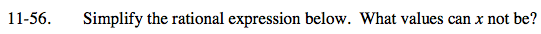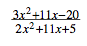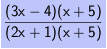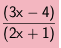### Home > CAAC > Chapter 11 > Lesson 11.1.5 > Problem11-56

11-56.The denominator cannot be equal to zero. What value(s) of x would make the denominator zero?

( 2x + 1 )( x + 5 ) = 0

2x2 + 11x + 5 = 0

x ≠ −0.5 or −5

Factor both the numerator and the denominator.Look for 1.